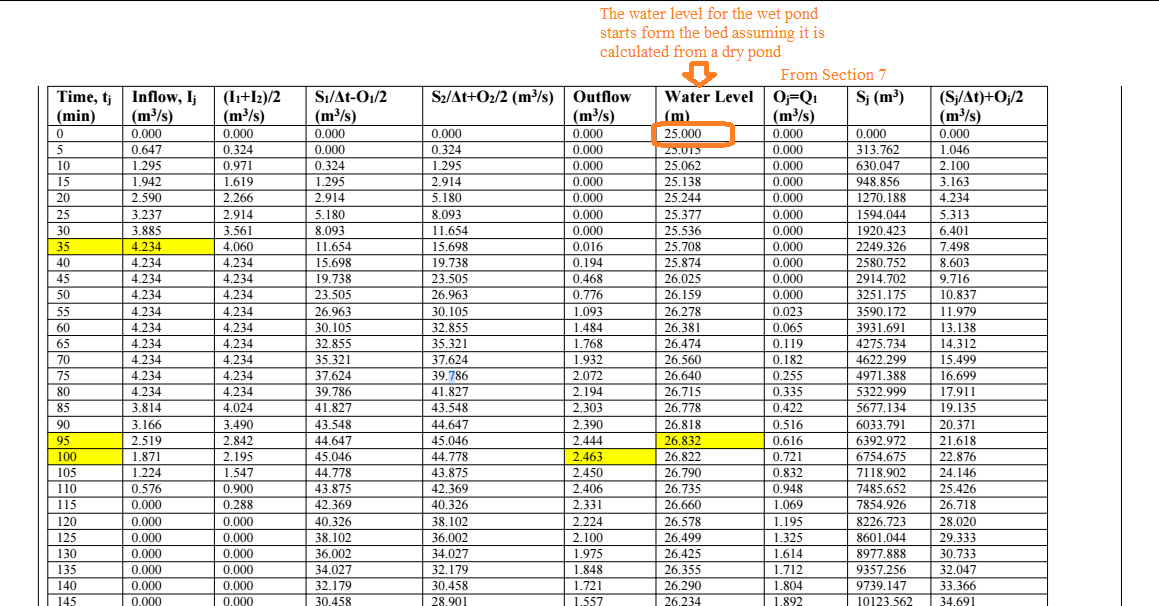603 - 5885 1250 (4 lines) info@mes100.com
Select Page

# Starting Water Level for Retention Pond

Latest MiTS 1 and 2 allows users to compute the analysis at any level of Starting Water Level (SWL), especially for the retaining pond. Default value is maintained as before which is the total of Invert Level (IL) and Height of bottom orifice (Invert Level + H).

Project reference

Normally a retention pond will have a starting water level which is equal to the H, the height of the minor orifice from the Pond Invert Level, and during raining time, the water will start at the H, and gradually go up. When the rain stops, the water will always maintain the level of H.

Thus, in the above example, the Starting Water level = 25 (invert level)+0.5 (H)=25.5m.

However, there are some situations whereby the Starting Water Level for a retention pond is not 25.5m, but 25m — at the Pond Invert Level,  such as when the very first instance when the retention pond is being put to use, and before there is any water that is filling in it.

Thus we are allowing the user to change the starting water level to cater for the situation whereby the calculation for a retaining pond needs to be done from a dry pond, and the routing is computed from the bed of the pond regardless of the H. If the analysis is required to be calculated from a dry pond, change the Starting Water Level (SWL) to be your invert level (ie 25 m based on this project file)

We believe that this flexibility also allows users to better understand the rises and falls of the flow depending on the starting water level.

Let’s take a look at how H and SWL can affect the result.

# Stage-Storage Relationship #

Do refer at Section 6 in the output analysis

Stage storage explains the storage volume at every stage of the elevation, which as in the project shows at every 0.05 m depth and cumulatively calculates on how much the pond size can cater. This analysis is calculated from the bed of the pond (invert level) regardless of the H and Start Water Level for.

The results for any SWL are the same since the analysis between the stage elevation and storage starts from the bed of the pond.

You can also refer to the Stage-Storage Relationship graph for a better understanding.

# Stage-Discharge Relationship#

Do refer at Section 7 in the output analysis

Stage-Discharge explains the discharge and the depth of water from the outlet at every ARI year given the size of the orifice.

This is where the H will influence the result, which is the discharge. The discharge will be calculated starting from the H regardless of the SWL, because the water will be retained below the H so there will be no discharge below that. Therefore the SWL won’t be affecting the calculation for the wet pond.

As example below, the H is located 0.5 m above the pond invert level. Result shows that the discharge starts right above the 0.5 m H which is at 25.55 m.

# Hydrologic Pond Routing#

Do refer at Section 8 in the output analysis

This section defines the relationships between the inflow and outflow hydrographs to duration. The storm durations will be routed in order to determine the critical duration that produces the highest water level and greatest discharge.

This section is affected by the H and SWL. The stage-discharge from the Section 7 will be considered in this pond routing which is affected by the H, therefore indirectly the result will be affected.

Some calculations need to be routed from a dry pond for a wet pond especially for new designs which acquire you to calculate from dry condition. Therefore, the SWL will be from the bed of the pond which is the invert level (i.e 25 m).

And as for the calculation for a wet pond assuming with a permanent retaining water depth, the calculation starts above the H (ie 25.5; invert level + H).

Wet Pond with 0.5 m H and 25 m SWL (routing is calculated from a dry pond):The calculation starts at 25 m, assuming that the routing is computed from a dry pond

Wet Pond with 0.5 m H and 25.5 m SWL (assuming the routing is calculated from a permanent retaining pond):The calculation starts at 25.5 m, assuming that the routing is computed from a permanent water level

Summary: